Technical Article

# Miller Frequency Compensation: How to Use Miller Capacitance for Op-Amp Compensation

June 12, 2019 by Dr. Sergio Franco

## Miller capacitance is commonly used in a method for operational amplifier frequency compensation.

Miller capacitance is commonly used in a method for operational amplifier frequency compensation.

In my previous articles, we discussed op-amp frequency compensation and one compensation method via shunt capacitance.

The frequency compensation technique in widest use today is called Miller frequency compensation, which we will explore in this article.

### What Is Miller Compensation?

Miller compensation is a technique for stabilizing op-amps by means of a capacitance Cƒ connected in negative-feedback fashion across one of the internal gain stages, typically the second stage.

### Utilizing Miller Compensation

Using the PSpice circuit of Figure 1, which was introduced in the previous article on frequency compensation, we obtain the magnitude/phase plots of Figure 2, showing that the presence of Cƒ causes the pole frequencies to split apart. Specifically, the higher the value of Cƒ is, the further apart the pole frequencies and, hence, the wider the intermediate frequency region of phase shifts close to –90°.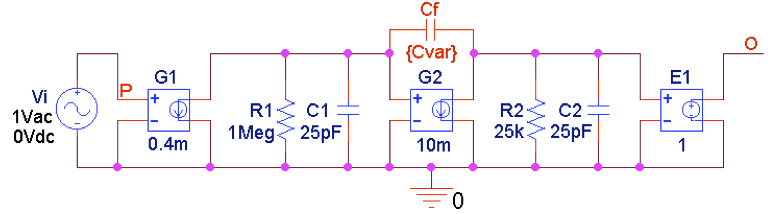##### Figure 1. PSpice circuit to plot the open-loop gain magnitude and phase for different amounts of Miller compensation.

Figure 3 provides an expanded view of the region of the crossover frequencies to facilitate a visual estimation of phase margins. Given a magnitude curve, (1) we identify the position of its crossover frequency ƒx on the 0-dB axis, (2) then we turn to the corresponding phase curve below, and (3) finally we read the phase shift ɸx at the right. Then the phase margin is ɸm = 180° + ɸx. For instance, for Cƒ = 8 pF, corresponding to the 4th curve after the Cƒ = 0 curve, we estimate ɸx ≈ –120°, so ɸm ≈ 60°.

Conversely, we can visually come up with a rough estimation for the value of Cƒ required for a given ɸm, and then refine Cƒ using the trial-and-error approach via PSpice. As an example, for ɸm ≈ 65.5°, which marks the onset of AC peaking, the above procedure yields Cƒ = 9.90 pF. The corresponding pole frequencies are measured to be 63.4 Hz and 12.2 MHz.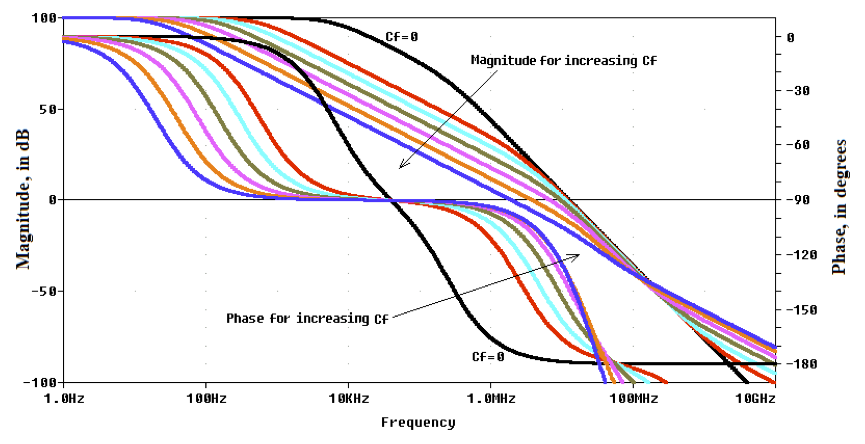##### Figure 2.  Magnitude/phase plots of the circuit of Figure 1 for different values of the compensating capacitance Cƒ: 0, 1 pF, 2 pF, 4 pF, 8 pF, 16 pF, and 32 pF.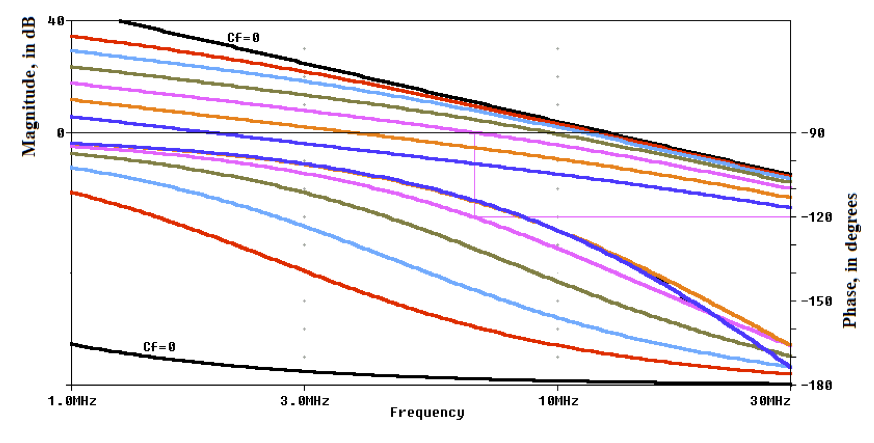##### Figure 3.  Expanded view of the area of the crossover frequencies of Figure 2.

Using the PSpice circuit of Figure 4 with Cƒ = 9.90 pF to provide Miller compensation, we get the plots of Figure 5, all of which are exempt from peaking!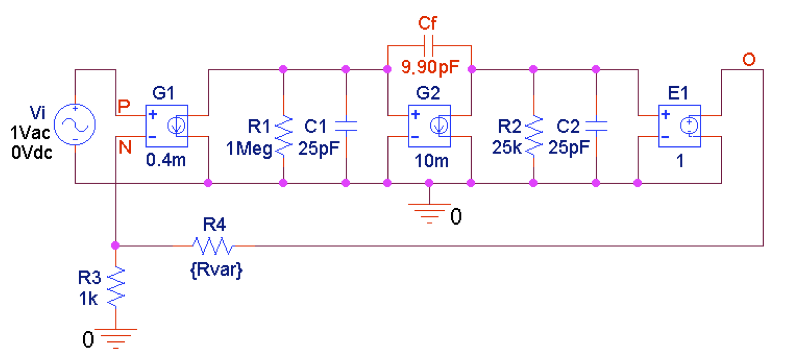##### Figure 4.  PSpice circuit to plot closed-loop gains in 20-dB steps determined by R4.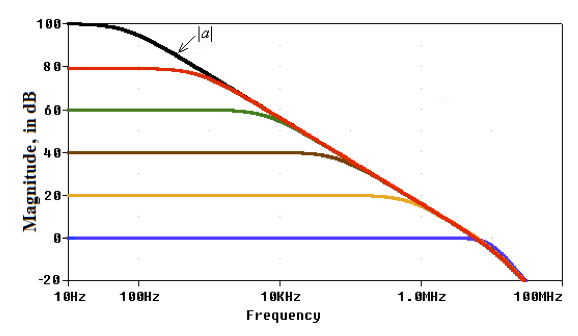### The Miller Effect for Capacitance

In the previous article on frequency compensation, we found that making the first pole dominant required a shunt capacitance of tens of nanofarads. Miller compensation, on the other hand, requires only picofarads

How come? The answer is provided by the Miller effect.

The Miller effect refers to the increase in equivalent capacitance that occurs when a capacitor is connected from the input to the output of an amplifier with large negative gain.

This concept is illustrated in Figure 6 for the capacitance case.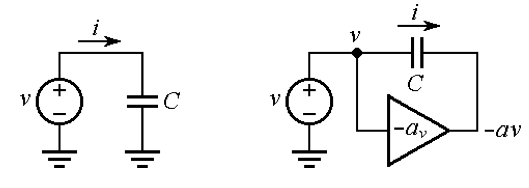##### Figure 6. Illustrating the Miller effect for capacitance.

In response to an applied voltage v, as in Figure 6(a), a capacitor C responds with the current $$i = C\frac{dv}{dt}$$. If we now connect the same capacitor C in feedback fashion across an inverting voltage amplifier with gain –av, as depicted in Figure 6(b), then the current becomes

### Miller Capacitance

The quantity CM in Equation 1 is referred to as the Miller capacitance and is calculated as follows

#### $$C_{M}=(1+a_{v})C$$

##### Equation 2. The Miller capacitance

In words, the feedback capacitance C reflected to the input, gets multiplied by 1 + av. This makes it possible to synthesize large capacitances with relatively small physical capacitors.

With reference to the PSpice circuit of Figure 4, we have

CM = (1 + Gm2R2)Cƒ = (1 + 250)9.90 pF = 2.485 nF

The total capacitance seen by R1 is Ctotal = CM + C1 = 2.51 nF, so the dominant pole frequency is 1/(2πR1Ctotal) = 63.4 Hz, in agreement with the value measured above via PSpice.

A systematic analysis of pole splitting, which is beyond the scope of this article, indicates that with Miller compensation, the new pole frequencies are approximately related to the originals ƒ1 and ƒ2 as

#### $$f_{2(new)}≈f_{2}\times G_{m2}R_{2}\times \frac{C_{f}}{C_{1}+C_{f}(1+\frac{C_{1}}{C_{2}})}$$

##### Equation 3

where ƒ1 = 1/(2πR1C1) and ƒ2 = 1/(2πR2C2). Since ƒ2(new) is directly proportional andƒ1(new) is inversely proportional to the second-stage gain Gm2R2, it is apparent that the larger this gain is, the wider the pole separation for a given Cƒ. This is highly desirable because with a sufficiently high gain, the required Cƒ for a given phase margin can be kept small enough (not more than a few tens of picofarads) so it can be fabricated on-chip. Moreover, the smaller Cƒ is, the faster the op-amp dynamics, since the open-loop bandwidth, slew rate, and full power bandwidth are all inversely proportional to Cƒ.

### A Bit of History

The first integrated circuit (IC) op-amp to incorporate full compensation was the venerable µA741 op-amp (Fairchild Semiconductor, 1968), which used a 30-pF on-chip capacitor for Miller compensation. The open-loop gain characteristics of the µA741 macro model available in PSpice are shown in Figure 7.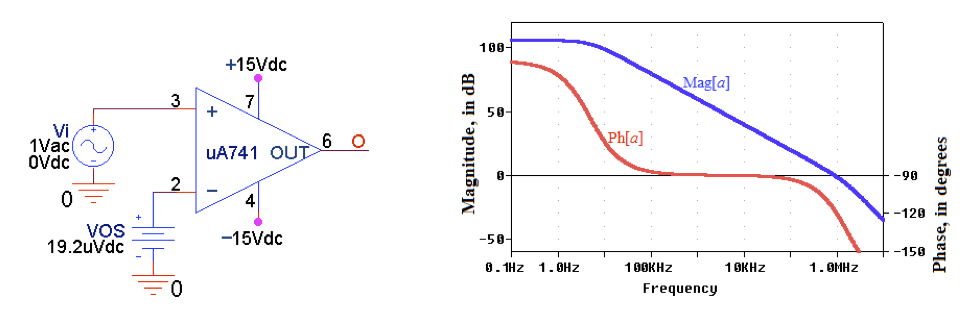##### Figure 7.  Plotting the open-loop gain a of the µA741 op-amp.

The magnitude curve crosses the 0-dB axis at ƒx = 888.2 kHz, where Ph[a] = –117°, for a phase margin of ɸm ≈ 63°.  Things go as if the µA741 had a second pole at ƒ2 = tan(ɸm)ƒx = 1.743 MHz.

Before the advent of the µA741, all IC op-amps had to be compensated externally by the user.  A popular uncompensated contemporary of the µA741was the LM301 (National Semiconductor), which offered the user three compensation options to meet different objectives: the single-pole compensation, the double-pole compensations, and the feedforward compensation

Even though the µA741 offered far less compensation flexibility than the LM301, the mA741 took off meteorically, most likely because many users were relieved of the oft-unpleasant task of providing external compensation without having a thorough understanding of its inner workings.

In the next article, we'll look at decompensated operational amplifiers.